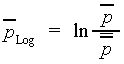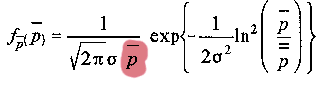### JPL's Wireless Communication Reference Website

#### Chapter: Wireless ChannelsShadowing is the effect that the received signal power fluctuates due to objects obstructing the propagation path between transmitter and receiver. These fluctuations are experienced on local-mean powers, that is, short-term averages to remove fluctuations due to multipath fading.

Experiments reported by Egli in 1957 showed that, for paths longer than a few hundred meters, the received (local-mean) power fluctuates with a 'log-normal' distribution about the area-mean power. By 'log-normal' is meant that the local-mean power expressed in logarithmic values, such as dB or neper, has a normal (i.e., Gaussian) distribution.

We distinguish between

• local means
denoted by a single overline
• area means
average over tens or hundreds of meters, to remove multipath fading and shadowing
denoted by a double overbar.
The received power PLog expressed in logarithmic units (neper), is defined as the natural logarithm of the local-mean power over the area-mean power, thusIt has the normal probability densitywhere s is the 'logarithmic standard deviation' in natural units. The standard deviation in dB is found from s = 4.34 s. For instance s = 6 dB shadowing is equivalent to s = 1.36. If we convert 'nepers' to 'watts', the log-normal distribution of received (local-mean) power is found.

Here the factor "1/local-mean power" occurs due to the conversion of the pdf of PLog to local-mean power.

Egli studied the error in a propagation model predicting the path loss, using only distance, antenna heights and frequency. For average terrain, he reported a logarithmic standard deviation of about s = 8.3 dB and 12 dB for VHF and UHF frequencies, respectively. Such large fluctuations are caused not only by local shadow attenuation by obstacles in the vicinity of the antenna, but also by large-scale effects (hills, foliage, etc.) along the path profile, which cause attenuation. Hence, any estimate of the area-mean power which ignores these effects may be coarse.

This log-normal fluctuation was called 'large-area shadowing' by Marsan, Hess and Gilbert. They measured semi-circular routes in Chicago , thus fixing distance to the base station, antenna heights and frequency, but measuring different path profiles. The standard deviation of the path loss ranged from 6.5 dB to 10.5 dB, with a median of 9.3 dB. This 'large-area' shadowing thus reflects shadow fluctuations if the vehicle moves over many kilometers.

In contrast to this, in most papers on mobile propagation, only 'small-area shadowing' is considered: log-normal fluctuations of the local-mean power are measured when the antenna moves over a distance of tens or hundreds of metres. Marsan et al. reported a median of 3.7 dB for small area shadowing. Preller and Koch measured local-mean powers at 10 m intervals and studied shadowing over 500 m intervals. The maximum standard deviation experienced was about 7 dB, but 50% of all experiments showed shadowing of less than 4 dB.

Mawaira of the Netherlands' PTT Research modelled large-area and small-area shadowing as two independent superimposed Markovian processes:

• 3 dB with coherence distance over 100 m, plus
• 4 dB with coherence distance 1200 m

## Implications for Cell Planning

Shadowing makes practical cell planning complicated. To fully predict local shadow attenuation, up-to-date and highly detailed terrain data bases are needed. If one extends the distinction between large-area and small-area shadowing, the definition of shadowing covers any statistical fluctuation of the received local-mean power about a certain area-mean power, with the latter determined by (predictable) large-scale mechanisms. Multipath propagation is separated from shadow fluctuations by considering the local-mean powers. That is, the standard deviation of the shadowing will depend on the geographical resolution of the estimate of the area-mean power. A propagation model which ignores specific terrain data produces about 12 dB of shadowing. On the other hand, prediction methods using topographical data bases with unlimited resolution can, at least in theory, achieve a standard deviation of 0 dB. Thus, the standard deviation is a measure of the impreciseness of the terrain description. If, for generic system studies, the (large-scale) path loss is taken of simple form depending only on distance but not on details of the path profile, the standard deviation will necessarily be large. On the other hand, for the planning of a practical network in a certain (known) environment, the accuracy of the large-scale propagation model may be refined. This may allow a spectrally more efficient planning if the cellular layout is optimised for the propagation environment.

###Exercise

The area-mean power corresponds to the logarithmic average of the received local mean power. Show that the linear average and higher-order moments of the local-mean power are of the form
```E[powern] = (area-mean power)n  exp(+n2s2/2)
```
The linear average is thus not identical to the area-mean power, except in the limiting case of s = 0.With shadowing, the interference power accumulates more rapidly than proportional to the number of signals. The accumulation of multiple signals with shadowing is a relevant issue in the planning of cellular networks.

## How do systems handle shadowing?

 System Countermeasure GSM Frequency planning and base station location Adaptive transmit power control DECT Base station location IS95 Cellular CDMA Power control. Power control in IS95 is also needed to achieve sufficient performance of the CDMA receiver. Base station locations Digital Audio Broadcasting Single frequency networks. Shadow fades are filled in by signals from other co-channel transmitters.